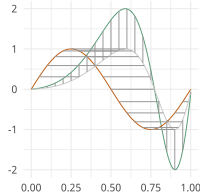# Elastic Functional Tolerance Bounds¶

Functional Tolerance Bounds using SRSF

moduleauthor:: J. Derek Tucker <jdtuck@sandia.gov>

tolerance.bootTB(f, time, a=0.5, p=0.99, B=500, no=5, parallel=True)[source]

This function computes tolerance bounds for functional data containing phase and amplitude variation using bootstrap sampling

Parameters: f (np.ndarray) – numpy ndarray of shape (M,N) of N functions with M samples time (np.ndarray) – vector of size M describing the sample points a – confidence level of tolerance bound (default = 0.05) p – coverage level of tolerance bound (default = 0.99) B – number of bootstrap samples (default = 500) no – number of principal components (default = 5) parallel – enable parallel processing (default = T) tuple of boxplot objects amplitude tolerance bounds ampbox object phase tolerance bounds phbox object alignment results fdawarp object
tolerance.mvtol_region(x, alpha, P, B)[source]

Computes tolerance factor for multivariate normal

Krishnamoorthy, K. and Mondal, S. (2006), Improved Tolerance Factors for Multivariate Normal Distributions, Communications in Statistics - Simulation and Computation, 35, 461–478.

Parameters: x – (M,N) matrix defining N variables of M samples alpha – confidence level P – coverage level B – number of bootstrap samples double tolerance factor
tolerance.pcaTB(f, time, a=0.5, p=0.99, no=5, parallel=True)[source]

This function computes tolerance bounds for functional data containing phase and amplitude variation using fPCA

Parameters: f (np.ndarray) – numpy ndarray of shape (M,N) of N functions with M samples time (np.ndarray) – vector of size M describing the sample points a – confidence level of tolerance bound (default = 0.05) p – coverage level of tolerance bound (default = 0.99) no – number of principal components (default = 5) parallel – enable parallel processing (default = T) tuple of boxplot objects alignment data from time_warping functional pca from jointFPCA tolerance factor
tolerance.rwishart(df, p)[source]

Computes a random wishart matrix

Parameters: df – degree of freedom p – number of dimensions double matrix